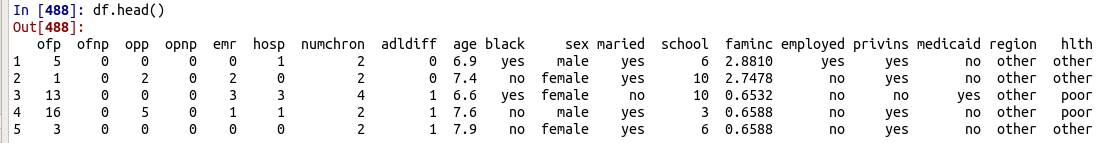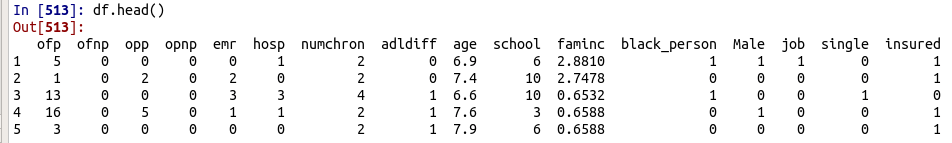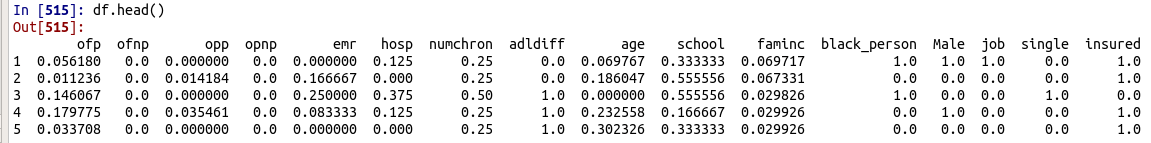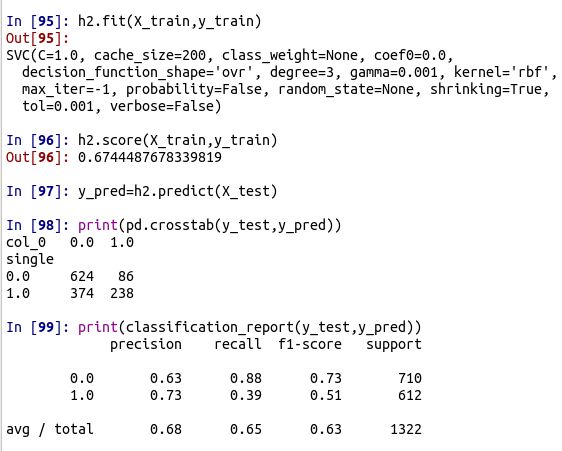# Support Vector Machines Classification with Python

Support vector machines (SVM) is an algorithm used to fit non-linear models. The details are complex but to put it simply  SVM tries to create the largest boundaries possible between the various groups it identifies in the sample. The mathematics behind this is complex especially if you are unaware of what a vector is as defined in algebra.This post will provide an example of SVM using Python broken into the following steps.

1. Data preparation
2. Model Development

We will use two different kernels in our analysis. The linear kernel and he rbf kernel. The difference in terms of kernels has to do with how the boundaries between the different groups are made.

Data Preparation

We are going to use the OFP dataset available in the pydataset module. We want to predict if someone single or not. Below is some initial code.

```import numpy as np
import pandas as pd
from pydataset import data
from sklearn import svm
from sklearn.metrics import classification_report
from sklearn import model_selection```

We now need to load our dataset and remove any missing values.

```df=pd.DataFrame(data('OFP'))
df=df.dropna()Looking at the dataset we need to do something with the variables that have text. We will create dummy variables for all except region and hlth. The code is below.

```dummy=pd.get_dummies(df['black'])
df=pd.concat([df,dummy],axis=1)
df=df.rename(index=str, columns={"yes": "black_person"})
df=df.drop('no', axis=1)

dummy=pd.get_dummies(df['sex'])
df=pd.concat([df,dummy],axis=1)
df=df.rename(index=str, columns={"male": "Male"})
df=df.drop('female', axis=1)

dummy=pd.get_dummies(df['employed'])
df=pd.concat([df,dummy],axis=1)
df=df.rename(index=str, columns={"yes": "job"})
df=df.drop('no', axis=1)

dummy=pd.get_dummies(df['maried'])
df=pd.concat([df,dummy],axis=1)
df=df.rename(index=str, columns={"no": "single"})
df=df.drop('yes', axis=1)

dummy=pd.get_dummies(df['privins'])
df=pd.concat([df,dummy],axis=1)
df=df.rename(index=str, columns={"yes": "insured"})
df=df.drop('no', axis=1)```

For each variable, we did the following

1. Created a dummy in the dummy dataset
2. Combined the dummy variable with our df dataset
3. Renamed the dummy variable based on yes or no
4. Drop the other dummy variable from the dataset. Python creates two dummies instead of one.

If you look at the dataset now you will see a lot of variables that are not necessary. Below is the code to remove the information we do not need.

```df=df.drop(['black','sex','maried','employed','privins','medicaid','region','hlth'],axis=1)This is much cleaner. Now we need to scale the data. This is because SVM is sensitive to scale. The code for doing this is below.

```df = (df - df.min()) / (df.max() - df.min())We can now create our dataset with the independent variables and a separate dataset with our dependent variable. The code is as follows.

```X=df[['ofp','ofnp','opp','opnp','emr','hosp','numchron','adldiff','age','school','faminc','black_person','Male','job','insured']]
y=df['single']```

We can now move to model development

Model Development

We need to make our test and train sets first. We will use a 70/30 split.

`X_train,X_test,y_train,y_test=model_selection.train_test_split(X,y,test_size=.3,random_state=1)`

Now, we need to create the models or the hypothesis we want to test. We will create two hypotheses. The first model is using a linear kernel and the second is one using the rbf kernel. For each of these kernels, there are hyperparameters that need to be set which you will see in the code below.

```h1=svm.LinearSVC(C=1)
h2=svm.SVC(kernel='rbf',degree=3,gamma=0.001,C=1.0)```

The details about the hyperparameters are beyond the scope of this post. Below are the results for the first model.The overall accuracy is 73%. The crosstab() function provides a breakdown of the results and the classification_report() function provides other metrics related to classification. In this situation, 0 means not single or married while 1 means single. Below are the results for model 2You can see the results are similar with the first model having a slight edge. The second model really struggls with predicting people who are actually single. You can see thtat the recall in particular is really poor.

Conclusion

This post provided how to ob using SVM in python. How this algorithm works can be somewhat confusing. However, its use can be powerful if use appropriately.